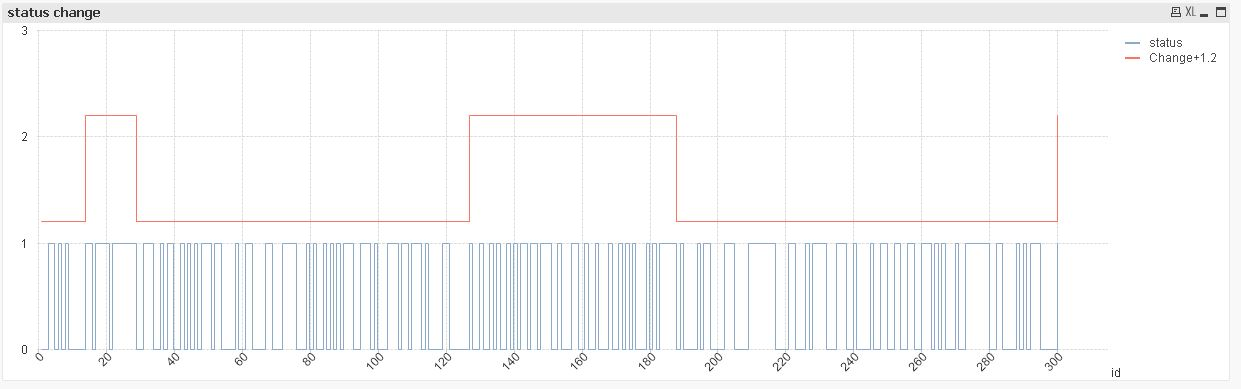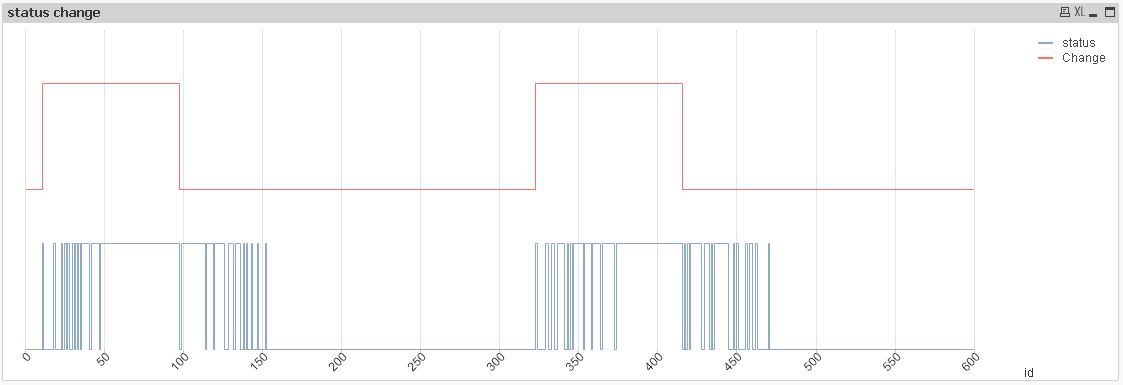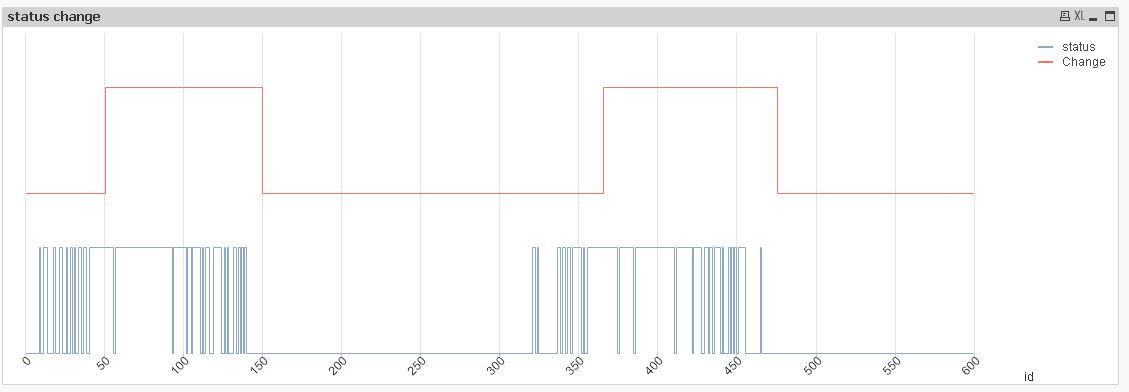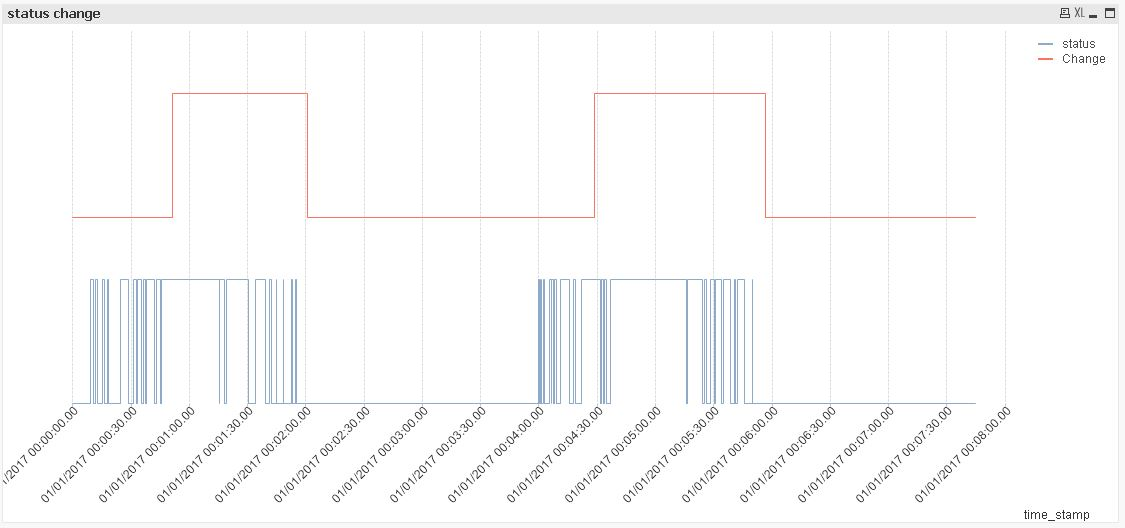QlikView Scripting

Discussion Board for collaboration on QlikView Scripting.

Announcements
Highlighted
New Contributor III

Range above function in load function.

Hi,

I am quite new to Qlik Sense, but I have some interesting problem, that I can't solve and I need your help.

I have a field that every 0.n second save 1 or 0 in database. So my data looks like a very long stream of 1 and 0.

Inside qlik sense I can detect when status has changed from 0 to 1, like this:

[id],

[time_stamp],

(If(status = '1' and Previous(status) = '0', 1, 0)) as Change;

My problem is, that there could be mild "errors", when status would change very quickly from 1 to 0 and back, like this:

[0,0,0,0,0,0,0,0,0,0,0,1,1,1,1,1,1,1,1,1,1,0,0,1,0,0,1,1,1,1,0,0,0,0,0,0,0,0]. I would like to detect this as only one change. (from 0 to 1)

So, my instinct was to check if previous n values are all zero and current value is 1. I saw that there is ABOVE function, but only for chart analysis, not for script function (load function). How to handle this? I would need something like:

[id],

[time_stamp],

(If(status = '1' and {sum of previous 20 status values} = '0', 1, 0)) as Change;

Even more, the second solution that I am looking for, and I would ask your help for, is to not rely on number of values, but rather on time stamp. So that function would be:

[id],

[time_stamp],

(If(status = '1' and {sum of all status values from time_stamp to time_stamp-2s} = '0', 1, 0)) as Change;

Tags (3)
1 Solution

Accepted SolutionsMVP & Luminary

Re: Range above function in load function.

I would rather go with something like this to identify the last changes and/or how many changes happened within the last n records:

[id],

[time_stamp],

if(id = previous(id), rangesum(peek('RowNoID'), 1), 1) as RowNoID,

ceil(peek('RowNoID') / 20) as RowNoIDCluster,

If(status <> Previous(status) = 0, peek('SumChange') + 1, peek('SumChange')) as SumChange,

If(status <> Previous(status), rowno(), peek('LastChange')) as LastChange;

and for grouping them to a certain time-period I would tend to round the timestamp appropriate with something like:

ceil(timestamp, 1/24/60/60)

to round up to full seconds.

It's not tested just some ideas and you will need to extend this kind of logic to a proper sorting and maybe also including some more checkings within the expressions - but I think the general logic will work. More to peek and previous could you find here: Peek() or Previous() ?

- Marcus

10 RepliesMVP

Re: Range above function in load function.

Hi,

one solution might be nested Previous() functions:table1:

status,

If(status = 1 and

Previous(status)=0 and

Previous(Previous(status))=0 and

Previous(Previous(Previous(status)))=0 and

Previous(Previous(Previous(Previous(status))))=0 and

Previous(Previous(Previous(Previous(Previous(status)))))=0,

1,

If(status = 0 and

Previous(status)=1 and

Previous(Previous(status))=1 and

Previous(Previous(Previous(status)))=1 and

Previous(Previous(Previous(Previous(status))))=1 and

Previous(Previous(Previous(Previous(Previous(status)))))=1,

0,

Alt(Peek(Change),status)

)

) as Change;

LOAD RecNo() as id,

Round(Rand()) as status

AutoGenerate 300;

but as your requested function already triggers on even the smallest glitches after a stable status period this is probably not what you really intended ?!?!

hope this helps nevertheless

regards

Marco

New Contributor II

Re: Range above function in load function.

If(STATUS = '1' and sum({<[TIMESTAMP] = {"<=\$(=[TIMESTAMP]))>=\$(=[TIMESTAMP]-'0:00:02')"}>}[VALUES])='0',1',0)) as Change

Hope this helps

New Contributor III

Re: Range above function in load function.

Yes, this was my first approach, but it make code really ugly, and can't be replaced with time period. Thank you anyway.

New Contributor III

Re: Range above function in load function.

Hi Henry,

Thank you for your answer, but I didn't understand it fully.

My first comment is, that as it seems to me, you can't use {} , \$, in load function. (At least your code, gave me an error at first {.)

My second question is who should this work? Sum all values where timestamp = timestamp >= timestamp - 2s?

I don't understand how would program know which timestamp is which.

Could you explain your solution a little bit further?MVP & Luminary

Re: Range above function in load function.

I would rather go with something like this to identify the last changes and/or how many changes happened within the last n records:

[id],

[time_stamp],

if(id = previous(id), rangesum(peek('RowNoID'), 1), 1) as RowNoID,

ceil(peek('RowNoID') / 20) as RowNoIDCluster,

If(status <> Previous(status) = 0, peek('SumChange') + 1, peek('SumChange')) as SumChange,

If(status <> Previous(status), rowno(), peek('LastChange')) as LastChange;

and for grouping them to a certain time-period I would tend to round the timestamp appropriate with something like:

ceil(timestamp, 1/24/60/60)

to round up to full seconds.

It's not tested just some ideas and you will need to extend this kind of logic to a proper sorting and maybe also including some more checkings within the expressions - but I think the general logic will work. More to peek and previous could you find here: Peek() or Previous() ?

- Marcus

New Contributor III

Re: Range above function in load function.

Thanks for your answer. I think it would be the right answer.

My new problem is that I can't use your calculated field

(If(id <> Previous(id) and id=1, peek('SumChange') + 1, 0)) as SumChange,

(If(id <> Previous(id), rowno(), peek('LastChange'))) as LastChange,

(If(SumChange=1 and LastChange - Previous(LastChange)>20, 1,0)) as numberOfLifts;

and I get error :

The following error occurred:MVP

Re: Range above function in load function.

Agreed, this code looks ugly.

Let me try another one:table1:

If(Peek(StatusCum)=Peek(StatusCum,-10) and status=1, 1,

If(Peek(StatusCum)=Peek(StatusCum,-10)+9 and status=0, 0,

Alt(Peek(Change),status))) as Change;

RangeSum(Peek(StatusCum),status) as StatusCum;

LOAD RecNo() as id,

Round((Rand()+Sin(RecNo()/50))/2) as status

AutoGenerate 600;

hope this helps

regards

MarcoMVP

Re: Range above function in load function.

another solution might be:table1:

If(StatChangeCum=Peek(StatChangeCum,-10) or id=1, status, Peek(Change)) as Change;

RangeSum(Peek(StatChangeCum),status<>Previous(status)) as StatChangeCum;

LOAD RecNo() as id,

Round((Rand()+Sin(RecNo()/50))/2) as status

AutoGenerate 600;

hope this helps

regards

MarcoMVP

Re: Range above function in load function.

or using a debounce time:table1:

If(time_stamp-ChangeTime>'00:00:05' or id=1, status, Peek(Change)) as Change;

If(status=Previous(status),Peek(ChangeTime),time_stamp) as ChangeTime;

LOAD RecNo() as id,

Timestamp(Alt(Peek(time_stamp)+fabs(NORMINV(Rand(),0,'00:00:01')),MakeDate(2017))) as time_stamp,

Round((Rand()+Sin(RecNo()/50))/2) as status

AutoGenerate 600;

hope this helps

regards

Marco